# 《芝麻街》人物赏析

### ELMo (Embeddings from Language Models)

#### 模型结构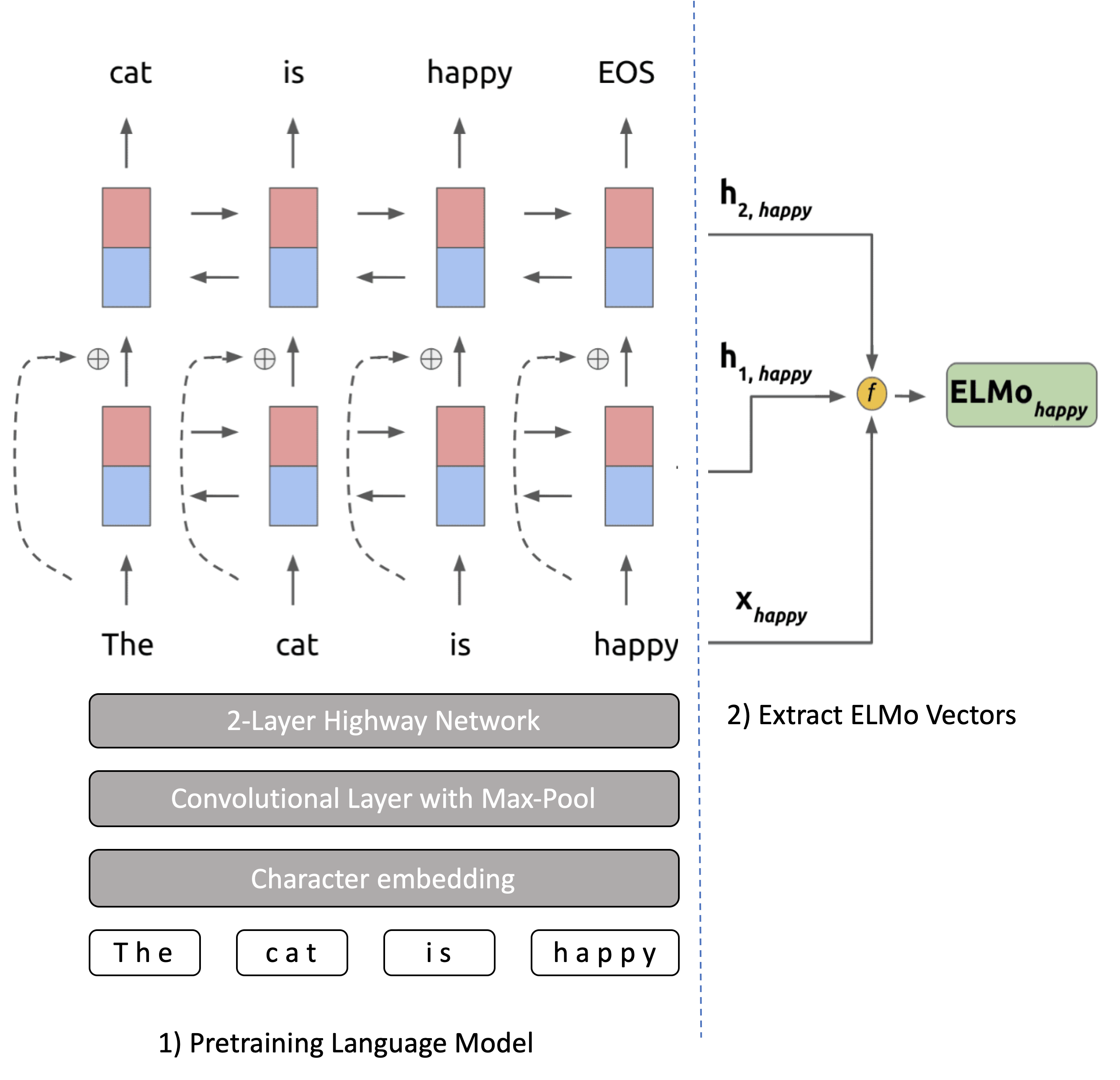$\sum_{k=1}^{N}(\log p(t_k|t_1,...,t_k-1;\Theta_x, \overrightarrow{\Theta}_{LSTM},\Theta_s)+\log p(t_k|t_{k+1},...,t_N;\Theta_x, \overleftarrow{\Theta}_{LSTM},\Theta_s))$$R_k=\{{x_k^{LM},\overrightarrow{h}_{k,j}^{LM},\overleftarrow{h}_{k,j}^{LM}|j=1,...,L}\} \\ ={\{h_{k,j}^{LM}|j=0,...,L\}}$$\textbf{ELMo}_k^{task}=E(R_k;\Theta^{task})=\gamma^{task}\sum_{j=0}^{L}s_j^{task}h_{k,j}^{LM}$

#### 实验分析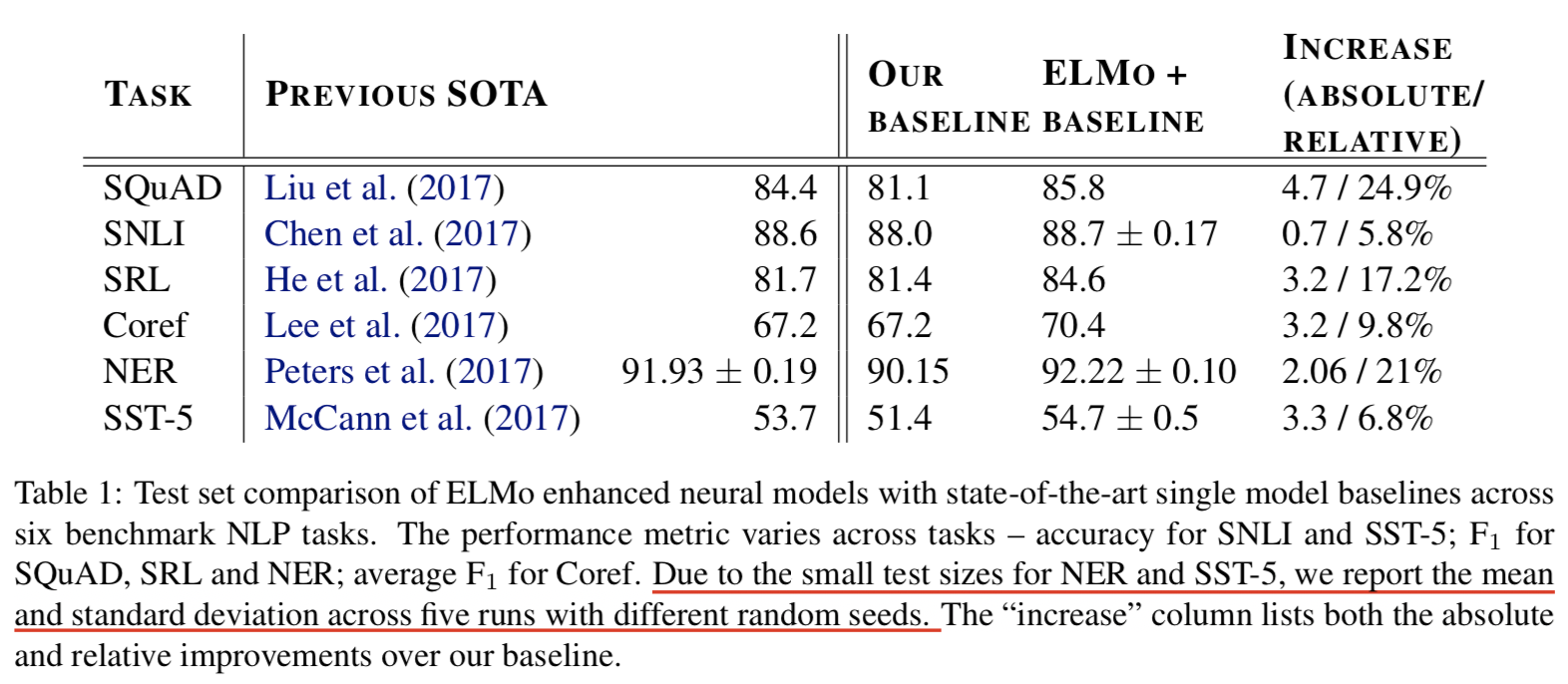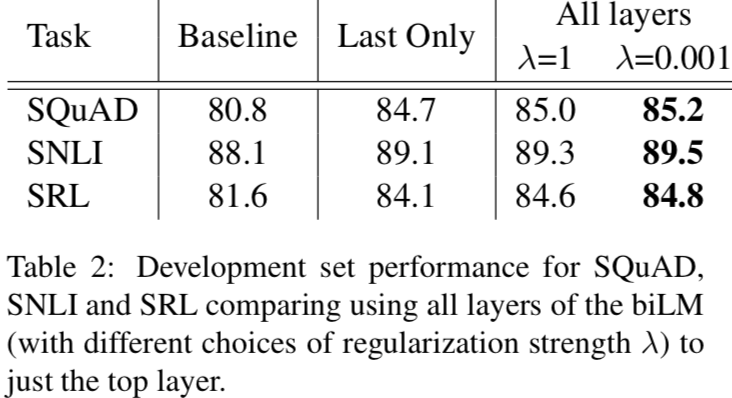$\lambda$是正则化控制项。对比列2和最后两列，显然综合多层要比直接利用最后1层好。而$\lambda = 1$实际上约束了各层的权重的可变性，轻微限制了模型的能力，$\lambda = 0.001$会更优秀些。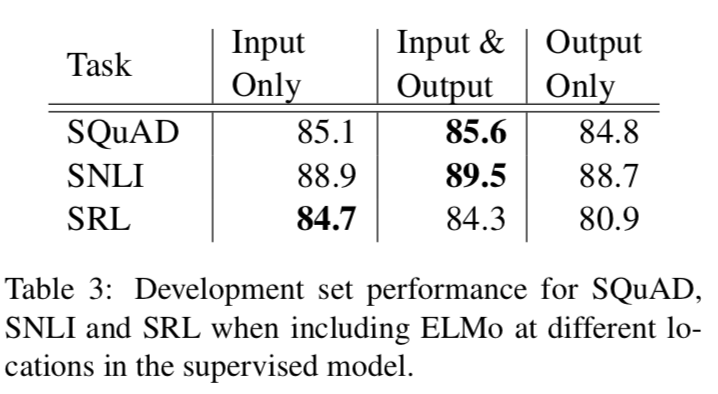具体拼接在哪里，还是得看具体任务的具体网络结构。注：这里前两个任务SQuAD和SNLI在biRNN之后是有attention层的，可以直接attend有用的信息。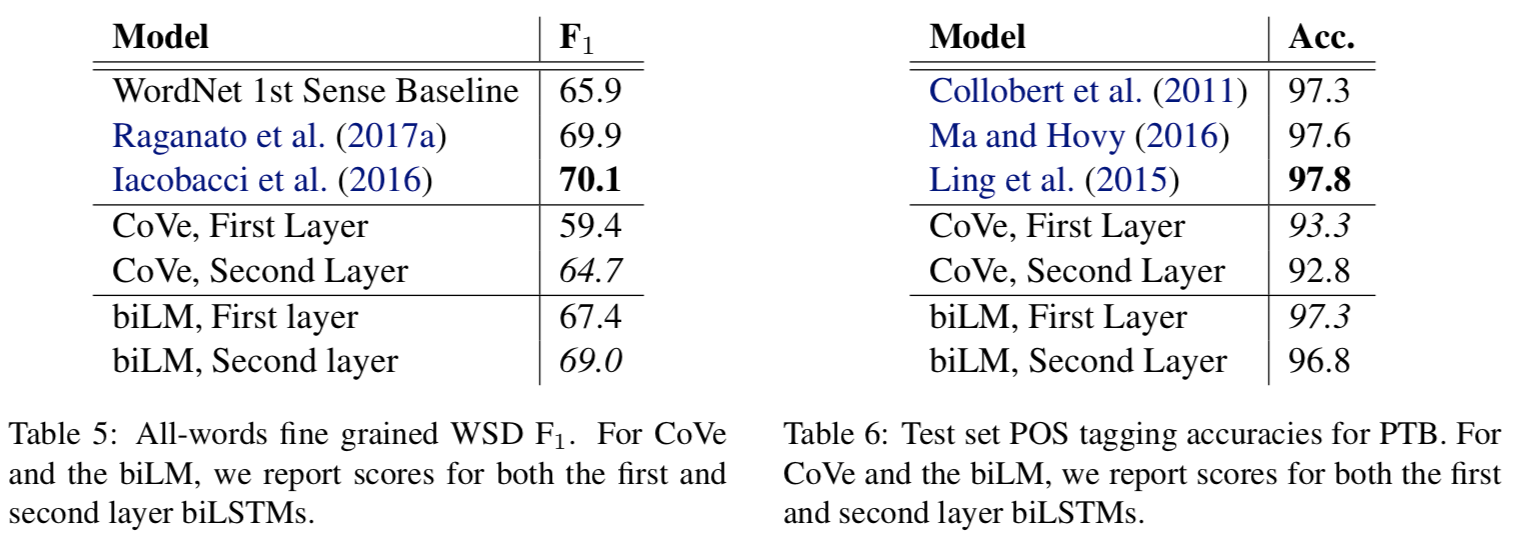ELMo的加入，对于下游监督任务的积极意义还有：

• 加速模型收敛（在SRL baseline模型中，达到最大Dev-F1需要486 epochs，加入ELMo后，在epoch 10就超过了baseline！）
• 在少标注数据上，提升巨大，如下图（1）
(1) Sample Efficiency (2) Weights Visualization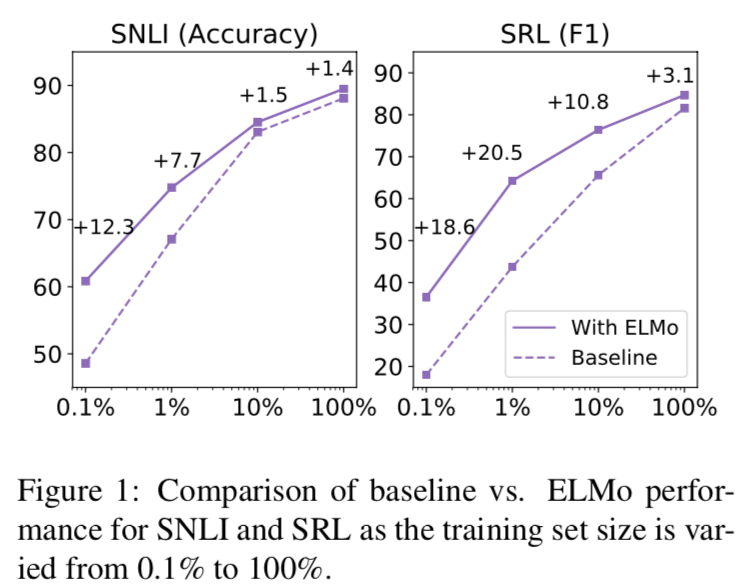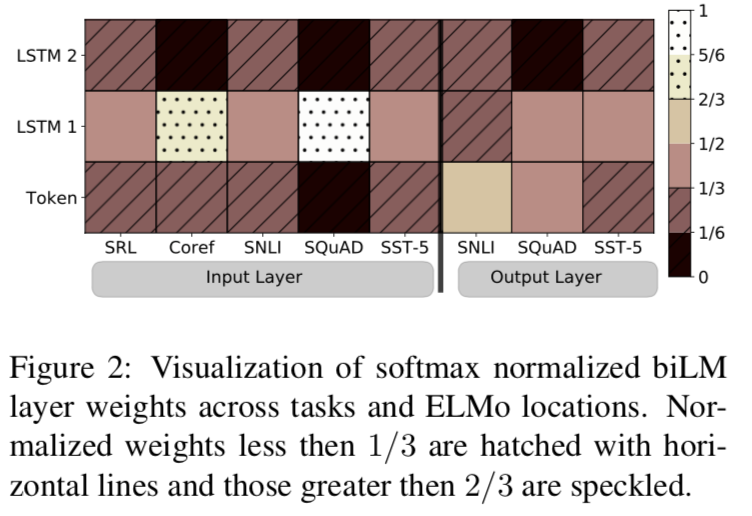### Language Representation / Understanding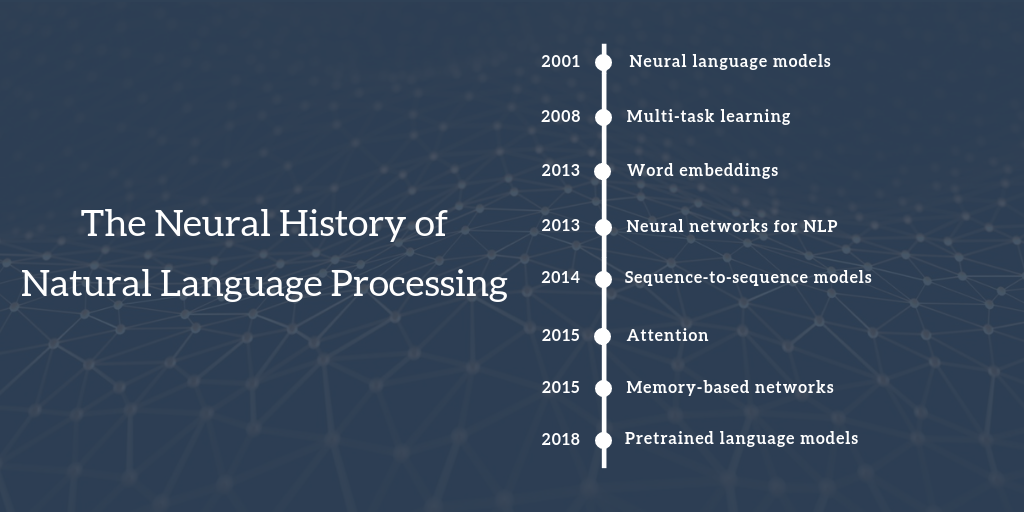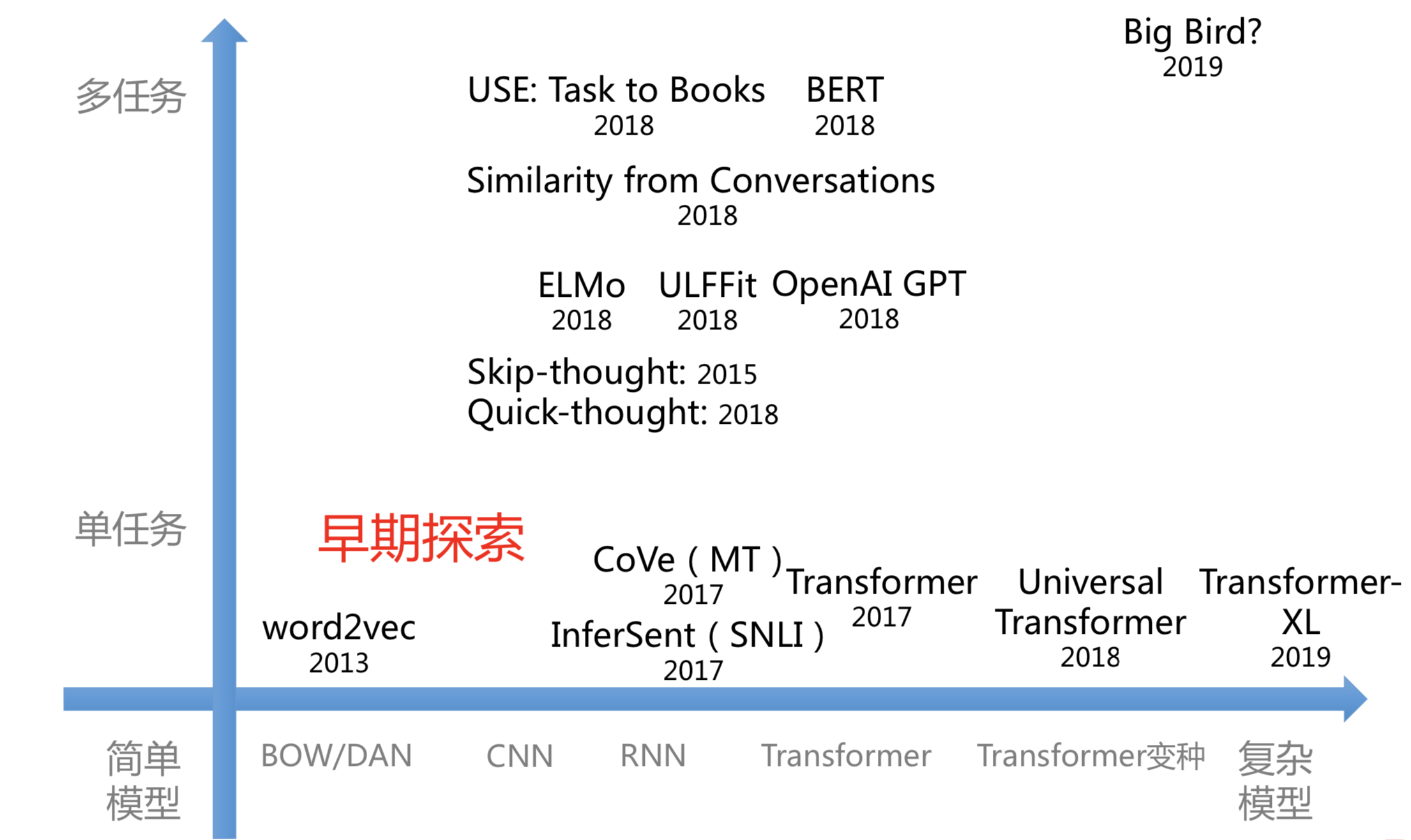NNLM (2003) 第一个神经网络语言模型。 词表示只是无心插柳的副产物。 启发了Word2Vec。
Word2Vec (2013) 用神经网络得到词向量表达。 思想简单，并提出两种高效优化算法。 无法解决一词多义问题，对上下文信息利用不够充分。 与后来的ELMo甚至BERT其实有千丝万缕的联系。
Glove (2014) 从词共现矩阵中得到的词向量表达。 训练速度极快，利用了全局信息。 同Word2Vec。
ELMo (2018) 通过双向语言模型学习上下文信息。 多义问题得到解决，融合双向context，语义语法信息更丰富。 LSTM特征抽取能力较弱，融合方式粗糙，预训练模型与下游模型脱轨。
GPT (2018) Transformer的解码器。 Pretrain+Finetune的训练范式。 自回归语言模型只能利用前向信息，长度受限。 Transformer XL、XLNet*

### Sesame Street 50th Anniversary

《芝麻街》（Sesame Street）是美国公共广播协会（2000年后由芝麻街工作室负责）制作播出的儿童教育电视节目，自1969年11月10日在美国公共电视台首播以来，已经陪伴全球140多个国家超过1亿的儿童健康快乐成长，荣获超过160次艾美奖

#### 50周年经典回顾

《芝麻街》播出50周年之际，让我们一起回顾一下那些难忘的经典画面（虽然我没有怎么看过，但是想想一档走过50年历史依旧备受欢迎的节目，还是觉得不可思议）：